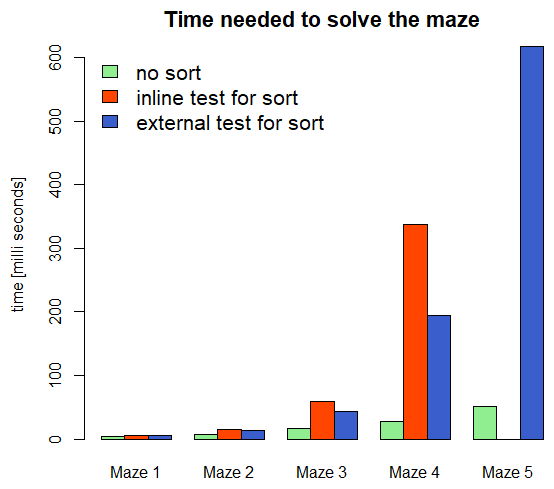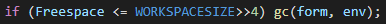# Tweetmaze Journey - An unexpected road to analyse memory usage

#1

During my first steps with µLisp I came across the Tweetmaze from David:

http://www.ulisp.com/show?1ANR

or here:

http://blog.mazelog.com/show?8WA

A really very nice example, from which I was able to learn a lot. In the end even much more than I thought at the beginning…

The codes from the website with the example mazes ran “out of the box”. To better understand how the algorithm works, I created two additional very simple mazes:

``````; A quite simple Tweetmaze
(defvar maze_1 '(2 7 3 4 1 1 5 0))

; A bit more difficult but still quite simple
(defvar maze_2 '(2 4 1 3 3 1 3 0))

; The "example Tweetmaze" from: http://www.lispology.com/show?ZXE
(defvar maze_3 '(7 3 4 7 7 3 7 2 5 8 4 9 3 4 2 0))

; The "slightly trickier Tweetmaze" from: http://www.lispology.com/show?ZXE
(defvar maze_4 '(2 6 4 9 5 7 2 4 8 4 3 4 2 7 5 0))
``````

David presented two functions for solving the mazes: One solution where the list of possible solutions is sorted before the function is recursively called again. The second solution does not use sorting, but only appends new solutions to the end of the solution list. Both functions find the same solutions, but they take different amounts of time. Sorting the list is of course a time consuming process - so nothing unexpected…

``````; Solution without sort
(defun solve-tweetmaze (maze &optional (queue '((0))))
(let ((goal (1- (length maze))))
(if (= (caar queue) goal)
(reverse (car queue))
(let* (    (top (pop queue))
(position (car top))
(right (+ position (nth position maze)))
(left (- position (nth position maze)))
)
(when (>= left 0) (setq queue (append queue (list (cons left top)))))
(when (<= right goal) (setq queue (append queue (list (cons right top)))))
(solve-tweetmaze maze queue)
))))

; Solution with sort
(defun solve-tweetmaze (maze &optional (queue '((0))))
(let ((goal (1- (length maze))))
(if (= (caar queue) goal)
(reverse (car queue))
(let* (    (top (pop queue))
(position (car top))
(right (+ position (nth position maze)))
(left (- position (nth position maze)))
)
(when (>= left 0) (setq queue (push (cons left top) queue)))
(when (<= right goal) (setq queue (push (cons right top) queue)))
(solve-tweetmaze maze (sort queue  (lambda (x y) (< (length x) (length y)))))
))))
``````

So far so good, but with a more difficult maze, it started to get interesting:

``````; A realy tricky maze
(defvar maze_5 '(8 9 1 2 2 4 4 2 2 2 9 1 2 6 1 0))
``````

The function without sorting provided the solution on my ESP32 after 51 milliseconds. The function with sorting, however, produced an error message:

Error: no room

The error “Error: no room” means that no more Lisp cells are available. Or in other words: That there is no more memory space available.

This was the moment when the simple trial of a sample code became a more intensive analysis of memory requirements and memory usage…

After a brief discussion with David, he suggested separating the test parameter of the sorting function because it might have something to do with giving a lambda expression as the test parameter to sort:

``````; Solution with "external" test parameter for sort
(defun lessthan (x y) (< (length x) (length y)))

(defun solve-tweetmaze (maze &optional (queue '((0))))
(let ((goal (1- (length maze))))
(if (= (caar queue) goal)
(reverse (car queue))
(let* (    (top (pop queue))
(position (car top))
(right (+ position (nth position maze)))
(left (- position (nth position maze)))
)
(when (>= left 0) (setq queue (push (cons left top) queue)))
(when (<= right goal) (setq queue (push (cons right top) queue)))
(setq queue (sort queue lessthan))
(solve-tweetmaze maze queue)
))))
``````

And indeed, with this modification, the function was able to solve this maze as well. It was even faster than the inline test version, which was not expected:But now I was getting curious and looked at the memory consumption of the function. When looking at how the function works, one notices that a list of possible solutions is created recursively. This list is extended with every call until a way to the goal is found.

So the list gets longer and longer and that means of course that more memory is needed. I then extended the function by a few outputs to have a closer look at the memory consumption over time:

``````(defvar loops 0)

(defun solve-tweetmaze (maze &optional (queue '((0))))
(let ((goal (1- (length maze))))
(print (incf  loops))
(princ  (concatenate 'string (princ-to-string (millis)) " " (princ-to-string (room)) " " (princ-to-string(length queue))))
(if (= (caar queue) goal)
(reverse (car queue))
(let* (    (top (pop queue))
(position (car top))
(right (+ position (nth position maze)))
(left (- position (nth position maze)))
)
(when (>= left 0) (setq queue (append queue (list (cons left top)))))
(when (<= right goal) (setq queue (append queue (list (cons right top)))))
(solve-tweetmaze maze queue)
))))

(setq loops 0)

(solve-tweetmaze maze_1)

n time room len
1 979479 7466 1
2 979484 7340 1
3 979489 7214 1
4 979494 7074 2
5 979499 6932 3
6 979504 6804 3
7 979509 6676 3
8 979514 6532 4
9 979519 6419 3
(0 2 5 4 3 7)
``````

Plotting the data ends up with the following graph:

It is clear to see how the length of the list increases and the available memory decreases. Also for the second ans third maze a similar picture can be seen:

It is interesting, however, that although the list is growing slowly, the memory is decreasing quite fast. This cannot be related to the pure length of the list and its memory requirements, but will probably have something to do with the recursive call.

How does it look like comparing the two functions (with sorting and without sorting)?

Even with the very simple maze, one can see that sorting requires more memory. Now I was very curious how it behaves with more difficult mazes:

Again, it is easy to see that sorting requires more memory space. The available memory decreases quickly. But with the 13th recursive call, the memory increases again. Suddenly there is more memory available again.

When evaluating the data from solving Maze 3, it can be seen that this phenomenon occurs more often:

What can be seen here is how the garbage collector (GC) works. The GC is called when the available memory has fallen below a certain threshold. The corresponding call can be found in the source code in the function eval():On my ESP32 the WORKSPACESIZE is = 8000; this means that the GC is called when the value is below 500. Until then all functions are allowed to “waste” the memory. Only then is it cleaned up.

When looking at the data from the call with Maze 5, it is easy to see that the GC is also called with the function without sorting. But this happens much later:

The sorting function therefore not only takes considerably more time, it also occupies considerably more memory. Since the GC also needs time for the cleanup, the whole function is even slower.

But what is the difference between the function with the test call within the sort command compared to the externally defined test? Here is the result:

This is a very interesting result and it also explains why the function with the externally defined test condition can solve the Maze 5 maze and the function with the inline defined test query cannot: It occupies too much memory too quickly.

The graph of the available memory looks really exciting when solving a maze which is considerably more complicated:

``````; A difficult maze
(defvar maze_6 '(8 8 6 2 2 2 7 5 4 2 5 1 5 1 9 0))
``````

By the way, my ESP32 needed 472610 milliseconds to calculate the solution, so 7 minutes and 52 seconds. I was actually faster solving the Tweetmaze “by hand”!